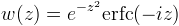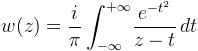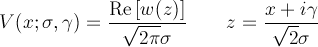Namespace:  Meta.Numerics.Functions
Assembly:  Meta.Numerics (in Meta.Numerics.dll) Version: 4.1.4Syntax
```public static Complex Faddeeva(
Complex z
)```

#### Parameters

z
Type: Meta.NumericsComplex
The complex argument.

#### Return Value

Type: Complex
The complex value of w(z).Remarks

The Faddeeva function w(z) is related to the error function with a complex argument.It also has an integral representation.For purely imaginary values, it reduces to the complementary error function (Erfc(Double)). For purely real values, it reduces to Dawson's integral (Dawson(Double)).

It appears in the computation of the Voigt line profile function V(x;σ,γ).Near the origin, w(z) ≈ 1. To accurately determine w(z) - 1 in this region, use the Erf(Complex) function. Away from the origin near the large negative imaginary axis, the magnitude w(z) increases rapidly and may overflow.

The image below shows the complex Faddeeva function near the origin, using domain coloring.See Also